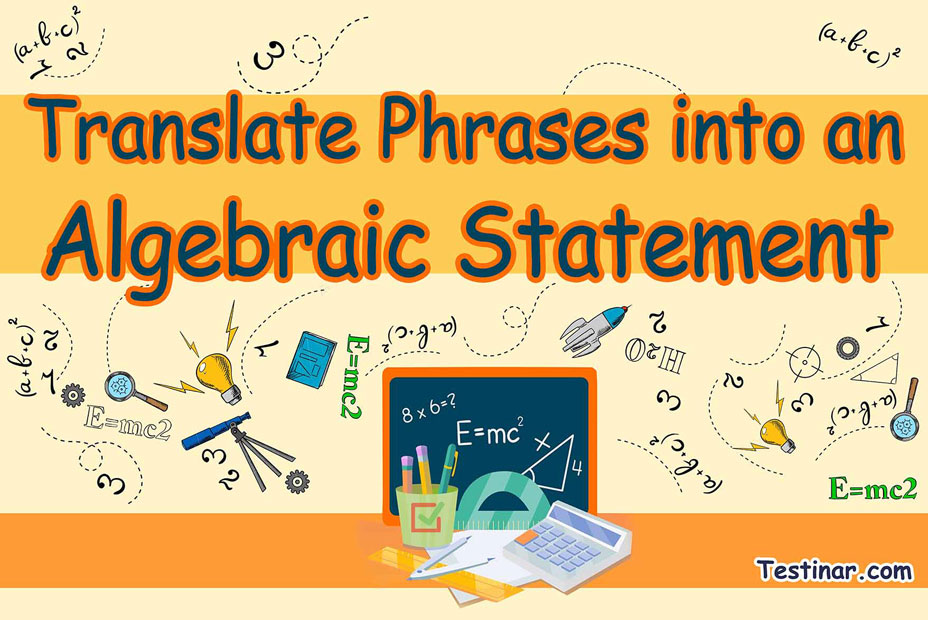## How to Translate Phrases into an Algebraic Statement?

### What are Algebraic Statements?

In mathematics, algebraic statements or expressions are defined as a combination of a certain number of terms (that might be variables or constants) which are separated by certain mathematical operations. Now, to be precise, these terms can be pure variables (like $$x^2, \ x^3$$), pure constants (like $$2, \ 9, \ 45$$, etc.) or even mixed terms with coefficients (like $$2x^2, \ 9x^3$$, etc.).
Also, if these algebraic statements contain an “equal to” sign in between, then the name changes to algebraic equation. Algebraic equations are statements where the left-hand side of the equation (basically the side left to the equal sign) has identical value to the right-hand side of the equation (the side right to the equal sign).
Now, sometimes certain phrases or terms may be used to chalk out an algebraic statement. These may include “plus”, “added to”, “subtracted from”, “times”, “divided by”, and so on.

### Algebraic Statements to Phrases

A variable expression or an algebraic expression is the combination of terms (constants, variables, or both) and is separated by arithmetic operations like addition, subtraction, multiplication, or division.
Some examples of expressions are: $$3x \ + \ 7, \ 7x^2 \ + \ 2x \ – 9, \ \frac{4}{9} \ x \ - \ 5$$, etc.

Let’s see the use of some variables in mathematical operations

• Addition: $$3 \ + \ x$$ ($$3$$ plus $$x$$)
• Subtraction: $$8 \ – \ a$$ ($$8$$ minus $$a$$)
• Division: $$7 \div x$$ ($$7$$ divided by $$x$$)
• Multiplication: $$7 \times b$$ ($$7$$ multiplied by $$b$$)

### Translating phrases into algebraic statements

• Addition: Addition can be translated as plus, more than, the sum of, etc. Ex: A number increased by $$15$$ would be $$x \ + \ 15$$.
• Subtraction: Subtraction can be translated as minus, less than, decreased, etc. Ex: A number decreased by $$15$$ would be $$x \ – \ 15$$.
• Multiplication: Multiplication can be translated as times, product, multiplied, etc. Ex: $$15$$ times a number would be $$15x$$.
• Division: Division can be translated as quotient, divided, ratio, etc. Ex: Thrice of a number divided by $$25$$ would be $$\frac{3x}{25}$$ .

### Exercises for Translate Phrases into an Algebraic Statement

1) A number increased by twenty.$$\ \Rightarrow \$$

2) A number decreased by eight.$$\ \Rightarrow \$$

3) five times the sum of a number and fifty.$$\ \Rightarrow \$$

4) two times the sum of a number and fifteen.$$\ \Rightarrow \$$

5) Twice a number divided by forty.$$\ \Rightarrow \$$

6) four times a number decreased by twenty-five.$$\ \Rightarrow \$$

7) A number divided by thirty-three.$$\ \Rightarrow \$$

8) The quotient of twenty-seven and a number.$$\ \Rightarrow \$$

9) The difference between sixteen and a number.$$\ \Rightarrow \$$

10) The sum of twenty-eight and a number.$$\ \Rightarrow \$$

1) A number increased by twenty.$$\ \Rightarrow \ \color{red}{x + 20}$$
2) A number decreased by eight.$$\ \Rightarrow \ \color{red}{x + 8}$$
3) five times the sum of a number and fifty.$$\ \Rightarrow \ \color{red}{5( x + 50)}$$
4) two times the sum of a number and fifteen.$$\ \Rightarrow \ \color{red}{2( x + 15)}$$
5) Twice a number divided by forty.$$\ \Rightarrow \ \color{red}{\frac{2x}{40}}$$
6) four times a number decreased by twenty-five.$$\ \Rightarrow \ \color{red}{4( x - 25)}$$
7) A number divided by thirty-three.$$\ \Rightarrow \ \color{red}{\frac{x}{33}}$$
8) The quotient of twenty-seven and a number.$$\ \Rightarrow \ \color{red}{\frac{27}{x}}$$
9) The difference between sixteen and a number.$$\ \Rightarrow \ \color{red}{16 - x}$$
10) The sum of twenty-eight and a number.$$\ \Rightarrow \ \color{red}{28 + x}$$

## Translate Phrases into an Algebraic Statement Practice Quiz

### ISEE Upper Level Mathematics Formulas

$6.99$6.99

### Praxis Core Math Practice Workbook

$25.99$14.99

### AFOQT Math Practice Workbook

$25.99$14.99

### The Most Comprehensive TASC Math Preparation Bundle

$79.99$36.99